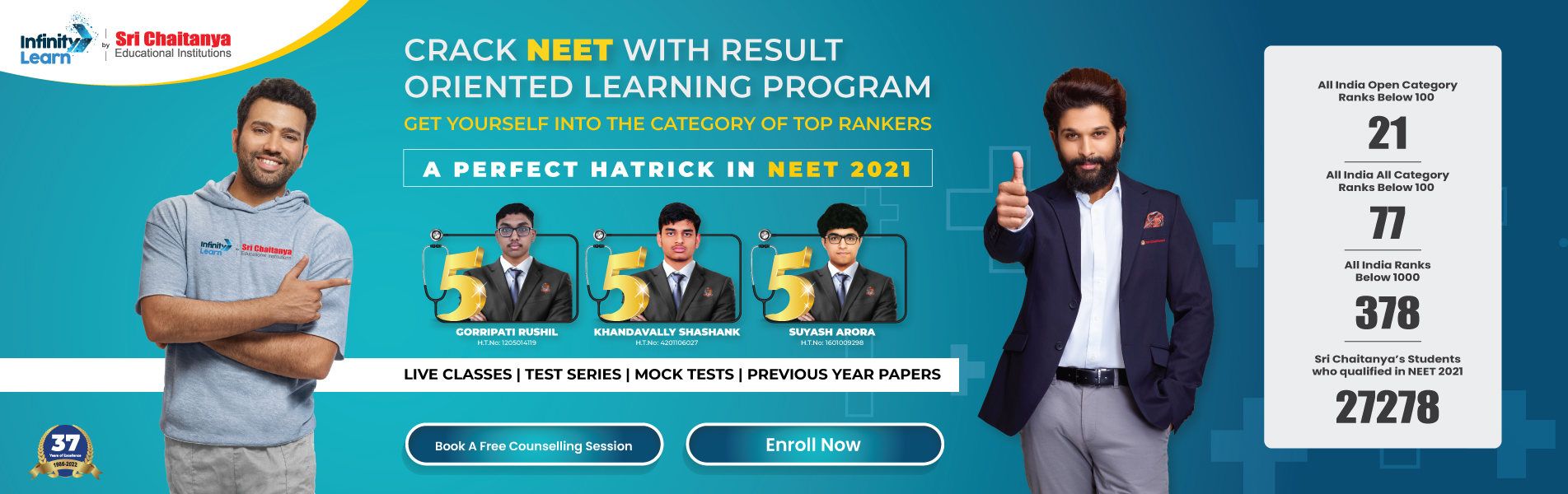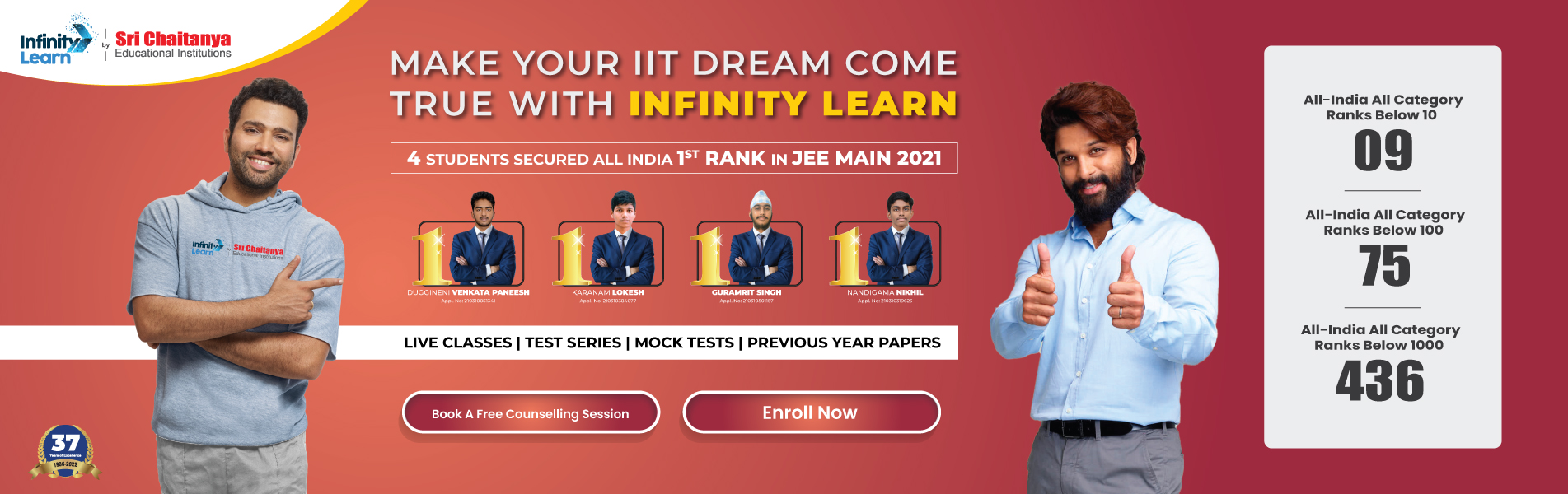NCERT Solutions for Class 11 Maths Chapter 6 Linear Inequalities Miscellaneous Exercise

# NCERT Solutions for Class 11 Maths Chapter 6 Linear Inequalities Miscellaneous ExerciseInfinity Learn NEET IIT-JEE
Infinity Learn NEET
banner-chaitanya-web

All miscellaneous exercise questions are answered in NCERT Solutions for Class 11 Maths Chapter 6 miscellaneous exercise Linear Inequalities. The principal studied in Maths chapter 11 are the foundation for NCERT Solutions for Class 11 miscellaneous exercise. This practice is crucial for both the CBSE Term II exam and competitive exams. To do well on your exam, download these NCERT Solutions and practice them offline.

Free IIT-JEE/NEET/NCERT/CBSE Material

Download 100,000+ FREE PDFs, solved questions, Previous Year Papers, Quizzes, and Puzzles directly to your Whatsapp account!

SOLUTIONS

NCERT Solutions for Class 11 Maths Chapter 6 Linear Inequalities Miscellaneous Exercise

Using the NCERT solutions for miscellaneous exercise Class 11 Maths is the best option for the CBSE students when they are preparing for their exams. This chapter includes many exercises. One of them is the miscellaneous exercise whose solutions are available in pdf format on this page. You can also download these solutions online from our website or app. Infinity Learn subject matter experts have designed solutions from the exercise with the utmost care and by keeping in mind all the guidelines by CBSE. Class 11 Students which have prepared with all the exercises given in this chapter can easily score well in the exams. By using these solutions, students came to know about the pattern of questions that are asked in the exam from this chapter and also know about the marks weightage of the chapter. So they can prepare well.

Along with this exercise, there are many number of exercises included in this chapter that contain innumerable questions as well. All these solutions are designed by subject matter experts as mentioned earlier. Hence all of these are considered as superior quality and students must refer to these solutions. To score well in the exam all these solutions must be practiced well and all other questions must be solved which are given next to it. Do not delay any more. Download the NCERT solutions for Class 11 Maths Chapter 6 miscellaneous exercise from Infinity Learn website now for better exam preparation. If you have the Infinity Learn app on your phone, you should download the same through the app as well. You can use these solutions online and offline as well

1. How to plot a linear inequality on a graph?

Ans. The equations should be arranged in a way such that ‘y’ is on the left side, while others are on the right. Next, learners need to plot the ‘y’ line (draw a continuous line when y<= or y>= and dotted line (when y< or y>). Finally, shade the area above the y line when (y> or y>=) or down the line (when y< or y<=).

2. Is class 11 Maths chapter 5 easy?

Ans. This chapter can become easy for students if they have the correct mindset. They need the correct guidance to get into the best path. The best guide today is Infinity Learn. Students can improve their scores by referring to these NCERT solutions. Students must use these NCERT Solutions to solve this chapter. The simplistic nature of the solution will help them to do the solutions with ease.

3. How many questions are covered in these NCERT Solutions?

Ans. This chapter is called ‘Linear Inequalities’. It consists of a total of three exercises with an additional miscellaneous exercise. NCERT Solutions of this chapter includes the following number of questions with solutions:

• Exercise 6.1 – 26 Questions
• Exercise 6.2 – 10 Questions
• Exercise 6.3 – 15 Questions
• Miscellaneous Exercise – 14 Questions

Join Infinity Learn Regular Class Programme!